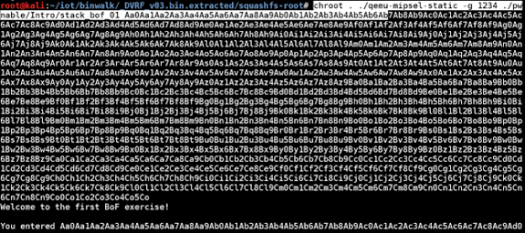# Do You Know Binaries?

10 Questions | Total Attempts: 46SettingsA binary number is a number expressed in the base-2 numeral system or binary numeral system. Think you already know your binaries? Take this quiz to prove yourself.

• 1.
What type of numerical system is used in Binaries?
• A.

Single-based

• B.

2-based

• C.

3-based

• D.

4-based

• 2.
Each digit in binary system is referred to as what?
• A.

Ones

• B.

Twos

• C.

Bits

• D.

None of the above

• 3.
In computing and telecommunications, what are binary codes used for?
• A.

Encoding data

• B.

Formatting data

• C.

Directing data

• D.

Rejecting data

• 4.
Binary numbers are also closely related to the methods used for ancient Egyptian multiplication.
• A.

False

• B.

True

• C.

Indecisive

• D.

None of the above

• 5.
In the binary system, each digit represents an increasing power of which of the following?
• A.

1

• B.

2

• C.

3

• D.

4

• 6.
Fractions in binary only terminate if the denominator has 2 or 0 as the only prime factor.
• A.

False

• B.

True

• C.

Only 0

• D.

None of the above

• 7.
Can addition, subtraction, multiplication and division can be performed on binary numerals?
• A.

Only multiplication and division

• B.

No

• C.

Yes

• D.

• 8.
To convert from a base-10 integer (normal numeric) to its binary equivalent, the number is divided by what?
• A.

10

• B.

1

• C.

2

• D.

4

• 9.
Binary system can be referred to as a system of numbers that use only two numbers. Which of these sets of numbers is correct?
• A.

0 and 1

• B.

1 and 2

• C.

2 and 4

• D.

None of the above

• 10.
All hacking tools consists of only binary combinations
• A.

True

• B.

False

• C.

None of the above

• D.

All of the above

Related TopicsBack to top